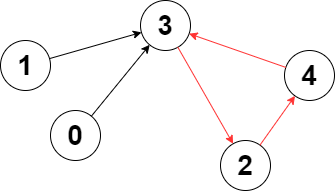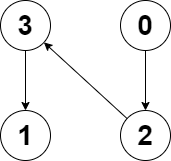2360. Longest Cycle in a Graph
Hard
2K
39

You are given a directed graph of `n` nodes numbered from `0` to `n - 1`, where each node has at most one outgoing edge.

The graph is represented with a given 0-indexed array `edges` of size `n`, indicating that there is a directed edge from node `i` to node `edges[i]`. If there is no outgoing edge from node `i`, then `edges[i] == -1`.

Return the length of the longest cycle in the graph. If no cycle exists, return `-1`.

A cycle is a path that starts and ends at the same node.

Example 1:```Input: edges = [3,3,4,2,3]
Output: 3
Explanation: The longest cycle in the graph is the cycle: 2 -> 4 -> 3 -> 2.
The length of this cycle is 3, so 3 is returned.
```

Example 2:```Input: edges = [2,-1,3,1]
Output: -1
Explanation: There are no cycles in this graph.
```

Constraints:

• `n == edges.length`
• `2 <= n <= 105`
• `-1 <= edges[i] < n`
• `edges[i] != i`
Accepted
63.2K
Submissions
124.9K
Acceptance Rate
50.6%

Seen this question in a real interview before?
1/4
Yes
No

Discussion (0)

Related Topics